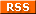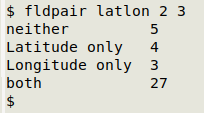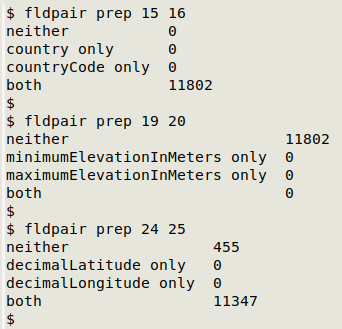For a full list of BASHing data blog posts see the index page.# How to do a both/neither/one/other tally - updated

A routine check I do on data tables is to see if paired fields are either both filled or both empty in each record. By "paired fields" I mean, for example, a latitude field and a longitude field. It doesn't make much sense to have a latitude without a longitude, or vice versa!

I wrote a command one-liner to tally up the "both filled", "both empty", "only the first filled" and "only the second filled" entries, and I've put the command in a function. To show the function at work, below is a tab-separated latitude/longitude table called "latlon". I've packed "latlon" in an HTML table with alternating lines to save space on this webpage. To get the 40 records in serial order, copy the table and paste it as a text file, then pass the file to sed to convert the third tab in each line to a newline:

sed 's/\t/\n/3' < file > latlon

 ID Latitude Longitude 001 153.4273 002 -29.4148 153.3504 003 -28.8635 153.5639 004 -29.5384 150.3841 005 -28.6467 147.6139 006 -29.0830 147.1818 007 008 -29.5489 152.3187 009 -28.4787 153.5510 010 -28.8002 153.5840 011 -29.0908 153.4327 012 -29.4000 153.3500 013 -29.3931 153.2331 014 -28.8635 015 016 -29.3696 017 -20.3500 148.8000 018 -27.2999 152.8861 019 020 -28.7551 021 -29.6001 152.1833 022 -28.8275 153.5329 023 -22.8167 149.8833 024 025 -28.1910 026 153.6139 027 0 0 028 -28.9334 149.3500 029 -27.0850 152.9762 030 -29.3640 150.9910 031 -26.9334 152.9500 032 -28.8502 153.0471 033 034 -28.9368 153.1744 035 -27.0237 152.9499 036 -29.3981 153.3686 037 153.1725 038 -29.1724 150.8508 039 -29.4869 153.3713

Here's my function, called "fldpair". It takes three arguments: filename, number of first field in pair, number of second field in pair. The commands are explained below.

UPDATE. In the first version of this blog post, "fldpair" didn't distinguish between an empty field and a field containing zero ("0"). The revised function only looks for genuinely empty fields.

fldpair() { awk -F"\t" -v one="\$2" -v two="\$3" 'NR==1 {x=\$one; y=\$two; next} (\$one!="") && (\$two!="") {both++} (\$one!="") && (\$two=="") {oneonly++} (\$one=="") && (\$two!="") {twoonly++} (\$one=="") && (\$two=="") {neither++} END {print "neither\t"neither"\n"x" only\t"oneonly"\n"y" only\t"twoonly"\nboth\t"both}' "\$1" | sed 's/\t\$/\t0/' | column -t -s \$'\t'; }

The result for "latlon":Some results for paired fields from a real-world table, "prep":The only "gotcha" I'm aware of for "fldpair" is that an empty field really has to be empty. If an apparently empty field actually contains a space or spaces of some kind, the AWK command will count it as filled. Please also note that the AWK command assumes the table is tab-separated.

awk -F"\t" -v one="\$2" -v two="\$3"
AWK is told what the table's field separator is (tab in this case), and AWK variables "one" and "two" are assigned to the shell command arguments 2 and 3, namely paired field 1 and paired field 2.

NR==1 {x=\$one; y=\$two; next}
The instruction for the first line of the table (the header) is to store the name of the first paired field in the variable "x" and the name of the second paired field in the variable "y". Once that's done, AWK moves to the next line.

(\$one!="") && (\$two!="") {both++}
If both of the paired fields are non-empty, the variable "both" is incremented by one.

(\$one!="") && (\$two=="") {oneonly++}
If the first paired field is non-empty but the second one is empty, increment "oneonly" by one.

(\$one=="") && (\$two!="") {twoonly++}
If the second paired field is non-empty but the first one is empty, increment "twoonly" by one.

(\$one=="") && (\$two=="") {neither++}
If both of the paired fields are empty, increment "neither" by one.

END {print "neither\t"neither"\n"x" only\t"oneonly"\n"y" only\t"twoonly"\nboth\t"both}'
With all lines processed, print the tallies for the four possible results with tab-separated labels. The "only" labels get their field names from "x" and "y".

"\$1"
This is the file which AWK has to process (the first argument for the function).

| sed 's/\t\$/\t0/'
AWK doesn't print anything if a tally is empty, so I pipe the AWK output to sed and convert any blank tallies to "0". An alternative would be to initialise the four incrementing variables to zero.

| column -t -s \$'\t'
I pipe the sed output to the column command to tidy the result.

Last update: 2020-08-19
The blog posts on this website are licensed under a